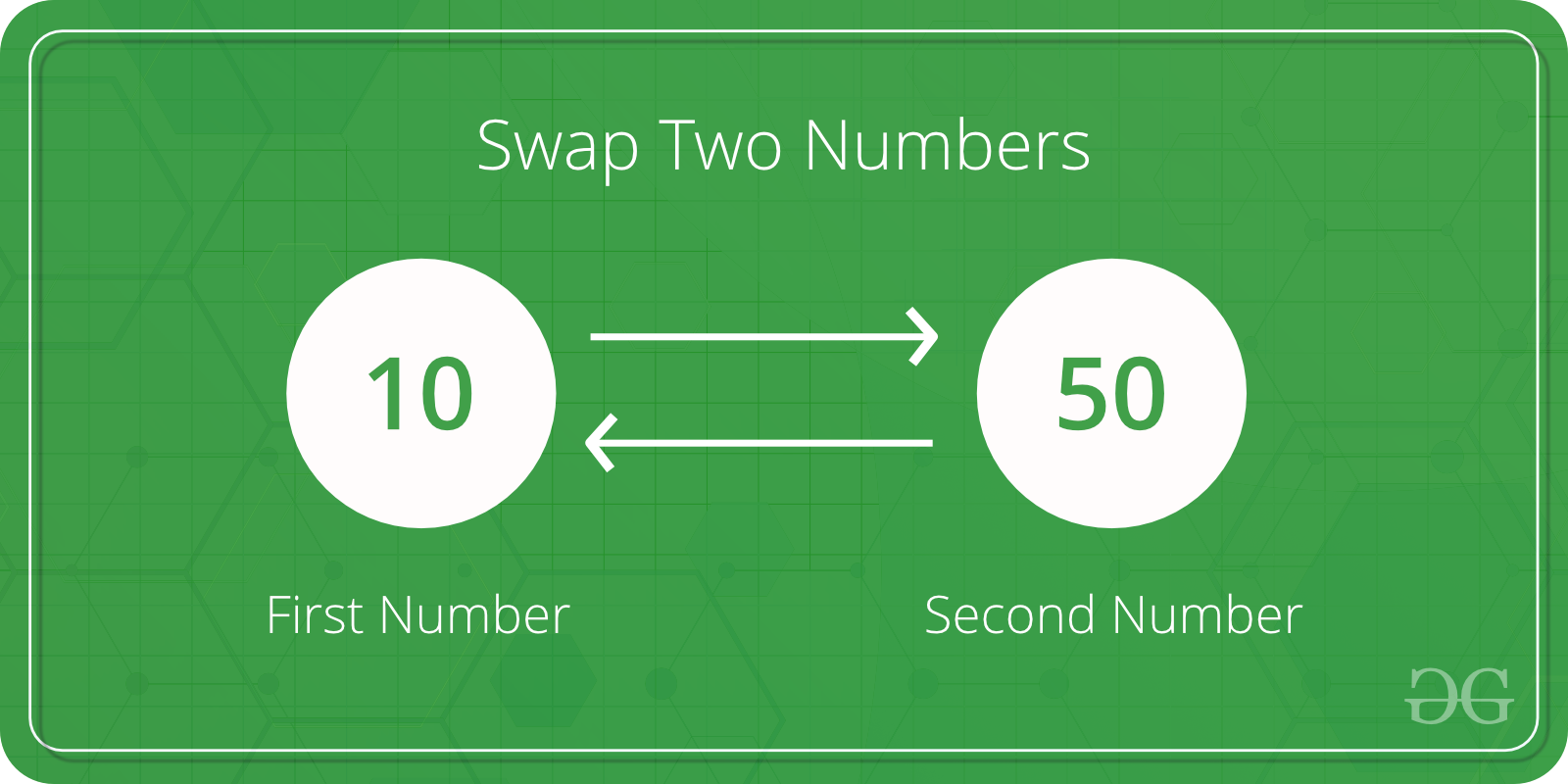Related Articles
C Program to Swap two Numbers
• Difficulty Level : Basic
• Last Updated : 07 May, 2020

Given two numbers, write a C program to swap the given numbers.

```Input : x = 10, y = 20;
Output : x = 20, y = 10

Input : x = 200, y = 100
Output : x = 100, y = 200
```## Recommended: Please solve it on “PRACTICE” first, before moving on to the solution.

The idea is simple

1. Assign x to a temp variable : temp = x
2. Assign y to x : x = y
3. Assign temp to y : y = temp

Let us understand with an example.

x = 100, y = 200

After line 1: temp = x
temp = 100

After line 2: x = y
x = 200

After line 3 : y = temp
y = 100

 `// C program to swap two variables``#include `` ` `int` `main()``{``    ``int` `x, y;``    ``printf``(``"Enter Value of x "``);``    ``scanf``(``"%d"``, &x);``    ``printf``(``"\nEnter Value of y "``);``    ``scanf``(``"%d"``, &y);`` ` `    ``int` `temp = x;``    ``x = y;``    ``y = temp;`` ` `    ``printf``(``"\nAfter Swapping: x = %d, y = %d"``, x, y);``    ``return` `0;``}`

Output:

```Enter Value of x 12

Enter Value of y 14

After Swapping: x = 14, y = 12 ```

How to write a function to swap?
Since we want the local variables of main to modified by swap function, we must them using pointers in C.

 `// C program to swap two variables using a ``// user defined swap()``#include `` ` `// This function swaps values pointed by xp and yp``void` `swap(``int` `*xp, ``int` `*yp)``{``    ``int` `temp = *xp;``    ``*xp = *yp;``    ``*yp = temp;``}`` ` `int` `main()``{``    ``int` `x, y;``    ``printf``(``"Enter Value of x "``);``    ``scanf``(``"%d"``, &x);``    ``printf``(``"\nEnter Value of y "``);``    ``scanf``(``"%d"``, &y);``    ``swap(&x, &y);``    ``printf``(``"\nAfter Swapping: x = %d, y = %d"``, x, y);``    ``return` `0;``}`

Output:

```Enter Value of x 12

Enter Value of y 14

After Swapping: x = 14, y = 12 ```

How to do in C++?
In C++, we can use references also.

 `// C++ program to swap two variables using a ``// user defined swap()``#include `` ` `// This function swaps values referred by ``// x and y,``void` `swap(``int` `&x, ``int` `&y)``{``    ``int` `temp = x;``    ``x = y;``    ``y = temp;``}`` ` `int` `main()``{``    ``int` `x, y;``    ``printf``(``"Enter Value of x "``);``    ``scanf``(``"%d"``, &x);``    ``printf``(``"\nEnter Value of y "``);``    ``scanf``(``"%d"``, &y);``    ``swap(x, y);``    ``printf``(``"\nAfter Swapping: x = %d, y = %d"``, x, y);``    ``return` `0;``}`

Output:

```Enter Value of x 12

Enter Value of y 14

After Swapping: x = 14, y = 12 ```

Is there a library function?
We can use C++ library swap function also.

 `// C++ program to swap two variables using a ``// user defined swap()``#include ``using` `namespace` `std;`` ` `int` `main()``{``    ``int` `x, y;``    ``printf``(``"Enter Value of x "``);``    ``scanf``(``"%d"``, &x);``    ``printf``(``"\nEnter Value of y "``);``    ``scanf``(``"%d"``, &y);``    ``swap(x, y);``    ``printf``(``"\nAfter Swapping: x = %d, y = %d"``, x, y);``    ``return` `0;``}`

Output:

```Enter Value of x 12

Enter Value of y 14

After Swapping: x = 14, y = 12 ```

How to swap without using a temporary variable?

Want to learn from the best curated videos and practice problems, check out the C Foundation Course for Basic to Advanced C.

My Personal Notes arrow_drop_up